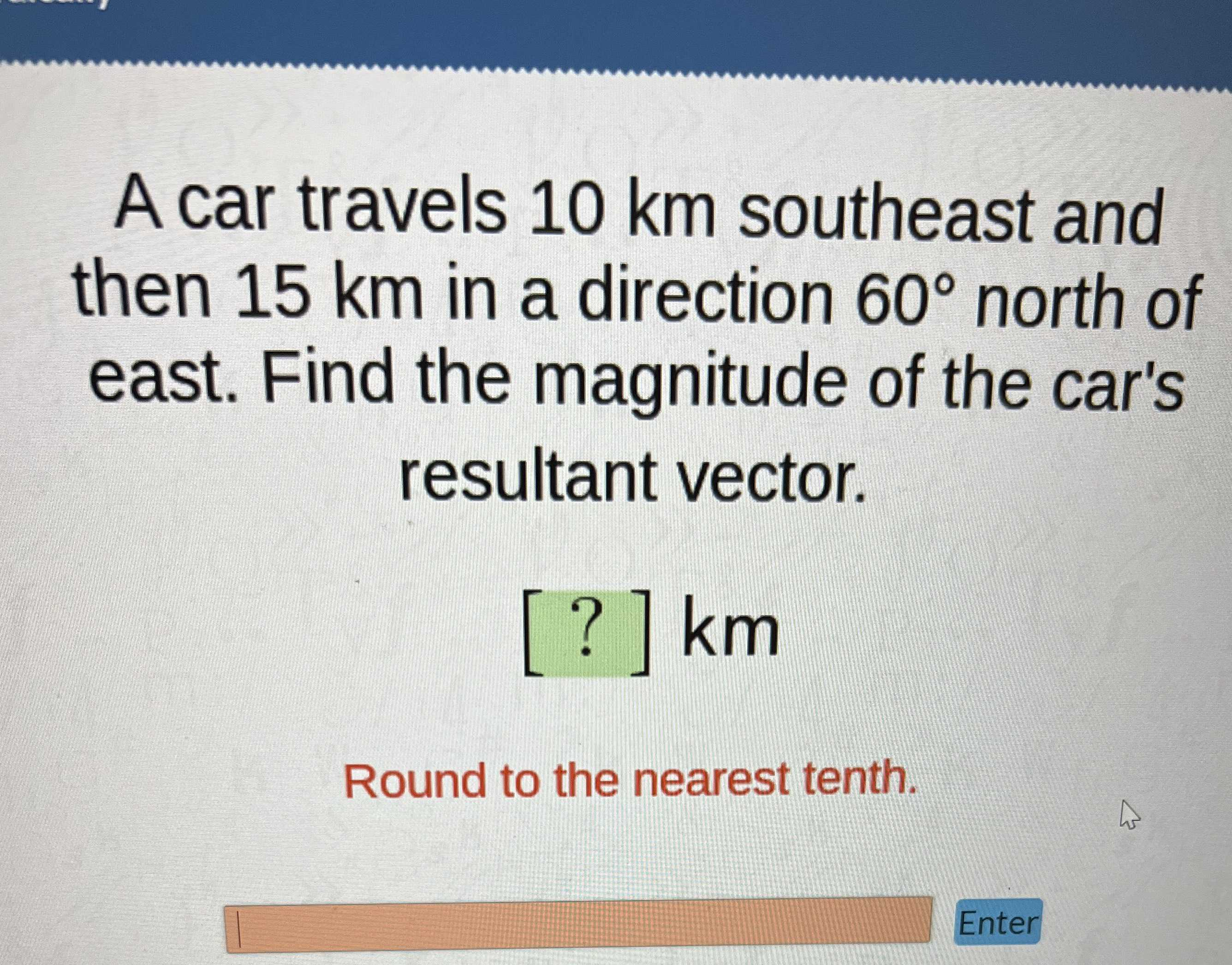### Still have math questions?

Trigonometry
QuestionA car travels $$10 km$$ southeast and then $$15 km$$ in a direction $$60 ^ { \circ }$$ north of east. Find the magnitude of the car's resultant vector.

$$[ ? ] km$$

Round to the nearest tenth.